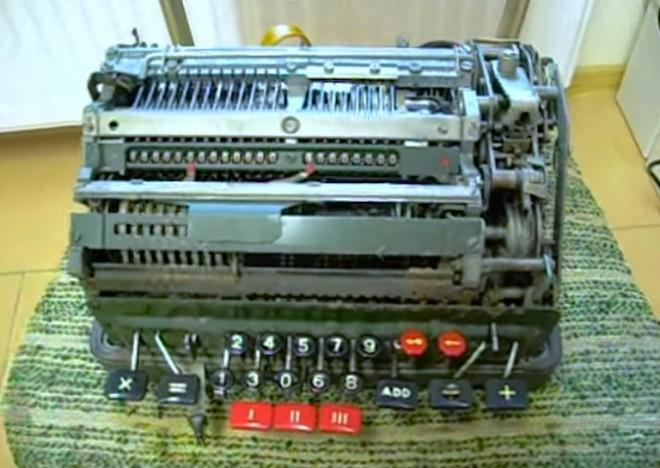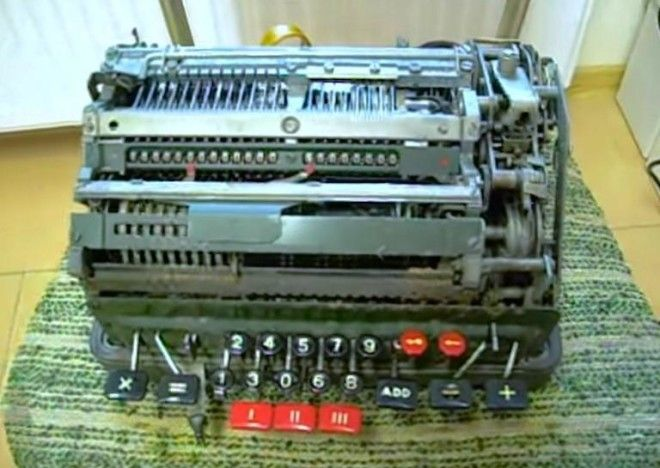# Old Calculator Nearly Explodes When it Divides by Zero

Try to perform the inexecutable equationWe all know that dividing by zero is impossible, but have you ever wondered what an old-timey mechanical calculator does when you try to perform the inexecutable equation. The formula of a number divided by zero is undefined as there is no number which multiplied by zero would equal a number not equal to zero. While that may have been confusing to some, just understand that when you divide by zero, bad things happen. As modern electronics have enabled calculators to simply output the answer of ERROR, typically dividing by zero wouldn’t be such a big deal.

However, with old mechanical calculators, it almost made them explode.

It’s likely that in order to stop this calculator from running itself into the ground, the operator would’ve had to have just pulled the plug. Can you imagine the shock and confusion of someone accidentally dividing by zero only to be met with your machine running out of control?

This calculator performs multiplication and division operations by using addition and subtraction as mechanically these are far simpler to run. So for example if you wanted to divide 12/4, the machine subtracts four from twelve and counts the number of times this occurred, being 3 in this case. Taking this mechanical principle and applying it to the equation of a number divided by zero, the machine essentially gets stuck in an infinite loop of trying to see how many times it can subtract 0 from a number. Each subtraction would result in the same number, thus starting the process all over again.For all you math whizzes out there, you may know that the mathematics behind calculations of dividing by zero is actually far more complex than simply saying it is infinity or undefined. Whether you’re good at math or not, the study of numbers and problem solving can be applied to all aspects of life, and is actually incredibly complex and interesting.

Source